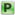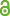Hauptmenü
• Autor
• Berkes, István
• Borda, Bence
• TitelOn the law of the iterated logarithm for random exponential sums
• Datei
• LicenceCC BY
• Zugriffsrechte• AbstractThe asymptotic behavior of exponential sums $$Σ^N_{k=1} exp(2πin_k α)$$ for Hadamard lacunary $$(n_k )$$ is well known, but for general $$(n_k )$$ very few precise results exist, due to number theoretic difficulties. It is therefore natural to consider random $$(n_k )$$ and in this paper we prove the law of the iterated logarithm for $$Σ^N_{k=1} exp(2πin_k α)$$ if the gaps $$n_{k+1} − n_k$$ are independent, identically distributed random variables. As a comparison, we give a lower bound for the discrepancy of $$\{n_k α\}$$ under the same random model, exhibiting a completely different behavior.